## Common Electric Circuits and Combination of Batteries

Common Electric Circuits and Combination of Batteries

In common electric circuits you can come across with, batteries, switches, and connection wires. We now examine the components of the simple circuit one by one.

1. Batteries: Device which supplies energy to the circuit is called battery. We show it in the circuit as;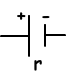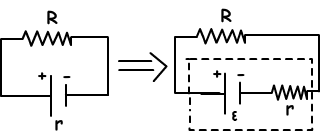Where; r is the internal resistance of the battery and it behaves like as it is combined to the circuit in series and ε is the electromotive force (EMF) of the battery.

EMF: It is the energy given by the battery to the unit charge when it passes from one end to the other end of the battery. If the battery gives W joule to a charge Q, then;

ε=W joule

We can say that, if the EMF of the closed circuit is known then EMF is directly proportional to the charge in the circuit.

W=ε.Q where Q=i.t

W=ε.i.t

units are;

W=joule, ε=volt, i=Ampere and t=s.

Combination of Batteries

Batteries can be combined in three ways, in series, in parallels and in opposite directions.

Batteries in Series: In this type of combination + end of the battery is connected to the – end of the other battery. Pictures given below show the examples of this type of combination.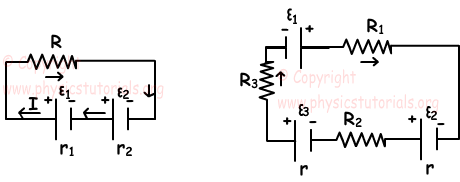Total EMF of the circuit is;

εeq123+...+εn

Total internal resistance of the batteries is;

req=r1+r2+r3+...+rn

Equivalent resistance of the circuit is;

Req=R+rt

Batteries in opposite directions:is the combination of the batteries  like shown in the figure below;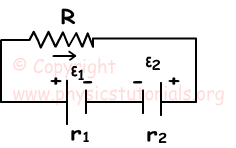In this type of circuits, battery having larger EMF supplies the energy of the circuit and battery having smaller EMF behaves like a resistor.Total EMF of the circuit is found by;

If ε1>ε2, εeq12

If ε1=ε2,εeq=0

If ε1<ε2, εeq=ε2-ε1

Total internal resistance of the batteries;

rt=r1+r2

Batteries in Parallel: In this type of circuits, batteries must be identical. Picture given below shows the example of batteries in parallel;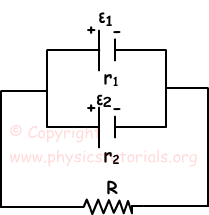Total EMF of the circuit;

εeq12=ε

Total internal resistance of the batteries is;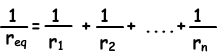Example: In the circuit given below, there are identical batteries. Voltmeter reads 60V when the switch is opened and 50V when the switch is closed. Find the internal resistance of the batteries.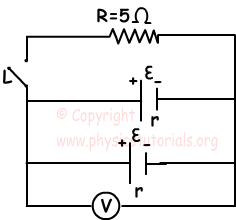60 volt is the EMF of the circuit since switch is opened. When we close switch we read 50 volt thus;

50volt=5Ω.i

i=10 ampere

Potential difference between the ends of the batteries, when the switch is closed, is;

50volt=60volt-10ampere.r/2 (r/2 is the equivalent resistance of the two batteries)

r=2Ω

Capacities of the batteries depend on the current passing through them. For example, look at the given circuits. In the first circuit, current i pass through the resistance and current i pass through each battery, however, in the second circuit current i again pass through the resistance however, current i/2 pass through the each battery. Thus, you can use A' and B' batteries longer than A and B batteries.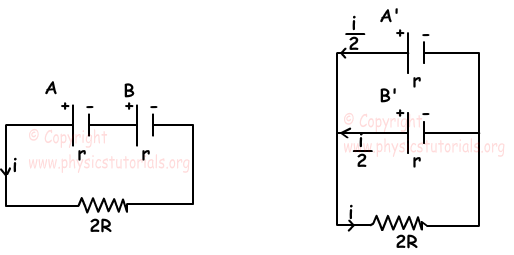Electric Current Exams and Solutions

Author: